Function Repository Resource:

# IdealCoinTossStatistics

Compute the probabilities and statistics associated with tossing a coin

Contributed by: Wolfram|Alpha Math Team
 ResourceFunction["IdealCoinTossStatistics"][n] computes probabilities and statistics associated with tossing n fair coins. ResourceFunction["IdealCoinTossStatistics"][n,h] computes probabilities and statistics associated with tossing n fair coins, of which h are heads. ResourceFunction["IdealCoinTossStatistics"][{n,w}] computes probabilities and statistics associated with tossing n coins having a probability of heads w. ResourceFunction["IdealCoinTossStatistics"][{n,w},h] computes probabilities and statistics associated with tossing n coins, of which h are heads. ResourceFunction["IdealCoinTossStatistics"][…,prop] returns property prop for the coin toss.

## Details and Options

ResourceFunction["IdealCoinTossStatistics"] returns an Association of properties with a subset of the following keys, depending on the input form:
For n>9, the "SampleSpace" property in the association is suppressed due to size.

## Examples

### Basic Examples (2)

Compute the probability and associated statistics of tossing 5 fair coins:

 In:=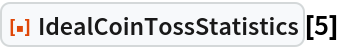Out=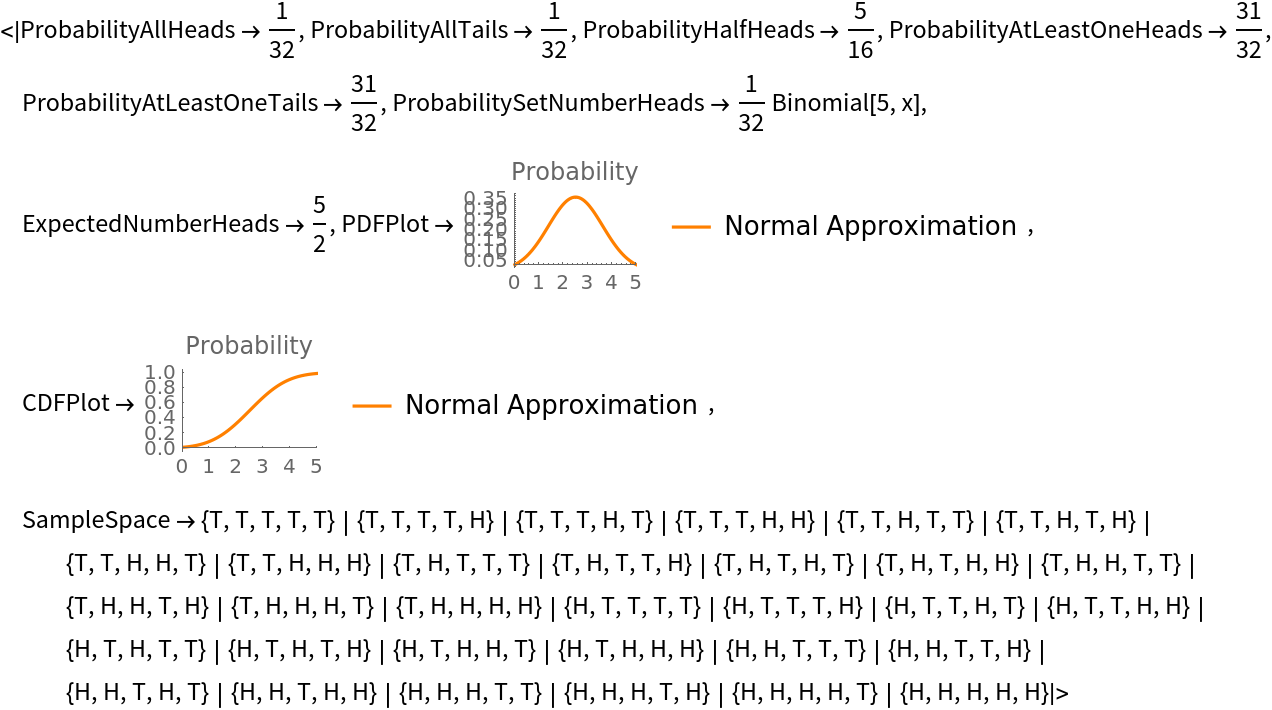Compute the probability and associated statistics of tossing 5 unfair coins:

 In:=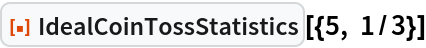Out=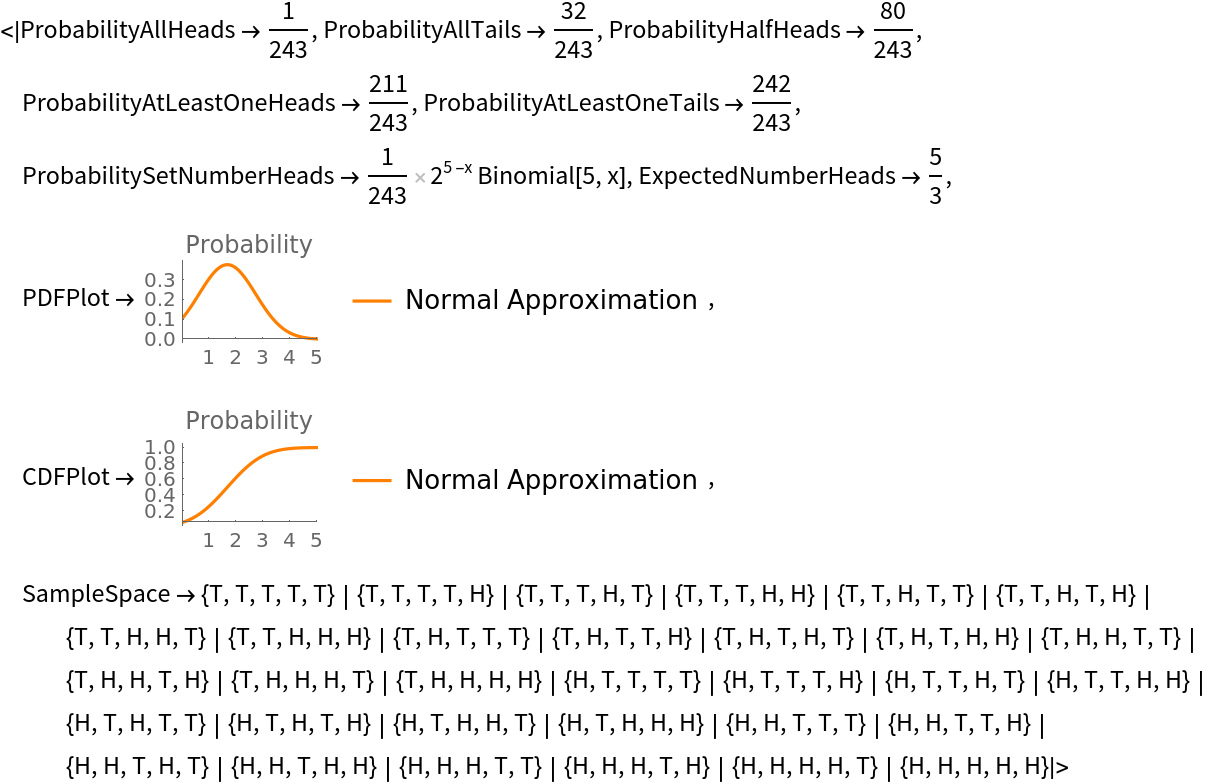Return only the probability density function plot:

 In:=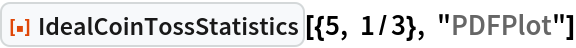Out=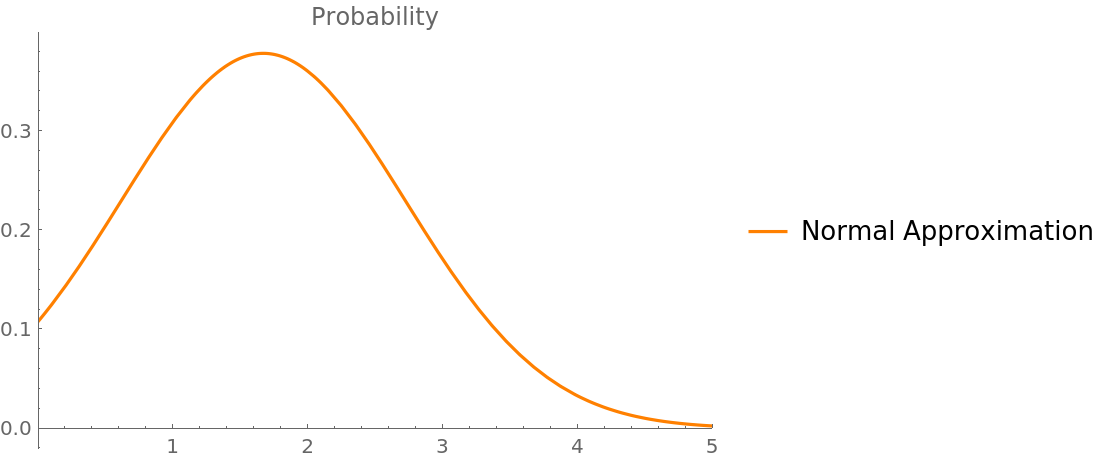### Scope (1)

Compute the probability and associated statistics of tossing 5 unfair coins, of which 3 are heads:

 In:=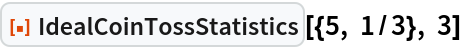Out=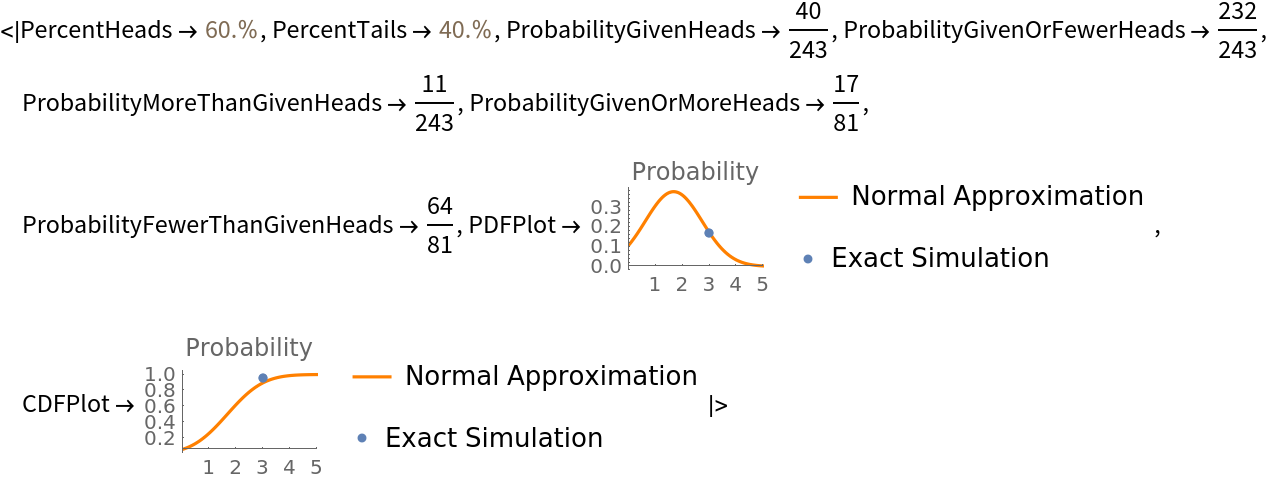### Properties and Relations (1)

To simulate a coin toss, use CoinTossSimulator:

 In:=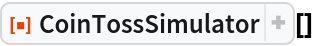Out=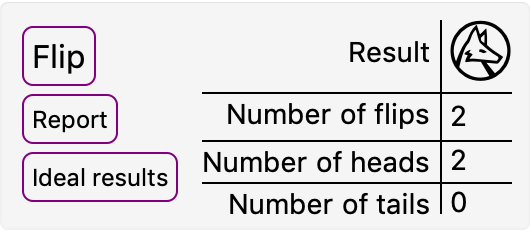## Publisher

Wolfram|Alpha Math Team

## Version History

• 2.0.0 – 23 March 2023
• 1.0.0 – 18 September 2020

## Author Notes

To view the full source code for IdealCoinTossStatistics, run the following code: